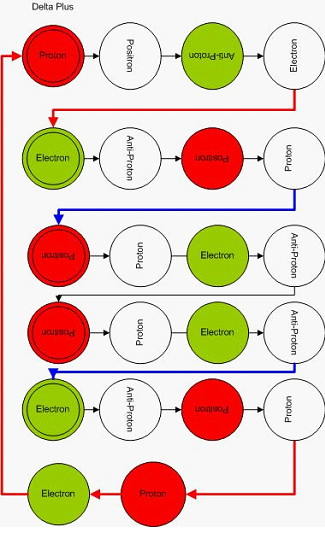# Hypergeometrical Universe

## The InterUniversal Geometry

In my prior posting about the ABC Conjecture I mentioned that in creating the assignment for the Hyperon Family, I came across the ABC conjecture.  I have to say that as many of the mystic mathematical associations with the Physical World, I wrote it half tongue-in-cheek.  Half because it takes a little more time than I have right now to investigate the extension of the validity of any association.  The other half is that I believe some if not all of those associations will be held in one way or another.

In my theory, particles can be represented by simple integers because they are multiples (in general) of the Fundamental Dilator. This makes my theory easy to map into Pure Mathematics.... :) All the other theories are cacophonies of "Quantum Numbers", thus necessarily multidimensional.  Quantum Numbers are used even while the reasoning for the word "Quantum" is not clear in those theories - no explicit quantizing process taking place anywhere.

### Associations:

• I mentioned that the charged hyperons mapped into Primes which make them related to the Riemann Hypothesis (that the poles of the Zetha should be primes).
• Any neutral hyperon should be a sum of two primes (two charged hyperons). This is the Goldbach's Conjecture..:)
• Neutral particles are Goldebach numbers...:)
• Every integer greater than 5 can be written as the sum of three primes, would indicate that any particle larger than five-dilators could have a three particle decaying path
• FIVE are the CRAZY DELTAS• Any Majorama particle is equal to 2^n.
• Any three particles dissociation process follows the ABC Conjecture.  This is simply because if they didn't the dissociation path would lead to a higher order many-body process or interaction.  The three numbers or particles should be co-primes, that is, they cannot be decomposed into a common particle otherwise the actual dissociation pathway would be the one for ABC divided by that common denominator.  So the family is to be created by bootstrapping coprimes of the Fundamental Dilator unit...:)

I liked that Shinichi  Mochizuki used the term InterUniversal Geometry..:)

If I am right, if one delves into describing the elasticity of space and the topology and taxonomy of particles, one will find that the ABC Conjecture has to do with volume in a number space. The volume of a number is the product of its primes

For instance

10=2*5 is two dimensional and has more volume than just 5.  Primes are unidimensional, thus are the axis of this infinite number space.

If you think about numbers as volumes in a number space, then ABC is a law of conservation of volumes.
Along the same lines, the Riemann Hypothesis or the zeros of a Zeta Function are the eigenvectors of a number space, each prime points to a different direction in it.

Similarly, the Goldbach conjecture is about even numbers being simple two dimensional retangles in the number space.

Similarly the Majorama particles being equal to 2^N gives rise to a number resonance in the Dilator Space..:)  1+3=4, 5+3=8 (5+3=8 is more stable than 1+7=8 mostly likely through entropic arguments....although most likely both pathways would be possible)...

This seems to talk about a unit of area in the number space.  So if you think about numbers as vector in a number space, the ABC conjecture can be thought as:
a)  rad(ABC) is the unit of volume ((AxB).C ) under the product ABC
b) C is the sum of two vectors in the number space, each with a length equal to A and  B (A and B is the same as Volume of A and Volume of B in the number space), although the number itself is a vector in that space.  This means that a number is both a scalar (volume) and a vector or a vector with a length equal to the volume... etc..:)
The ABC Conjecture looks to be like A+B -> C

There is a simple extension of this reasoning that might be clearer.

Cheers,

MP
Currently unrated

or